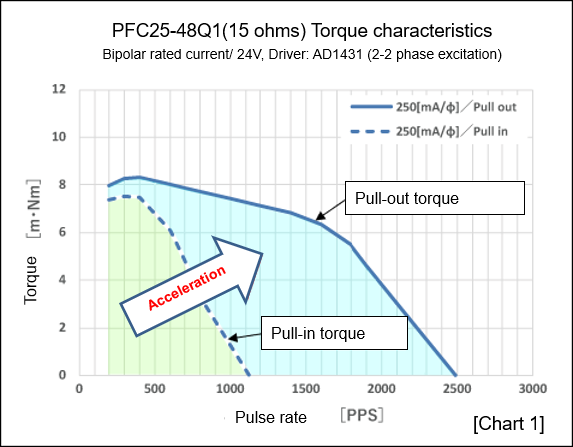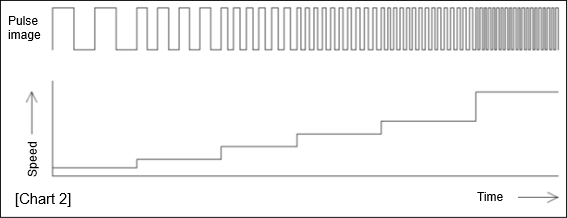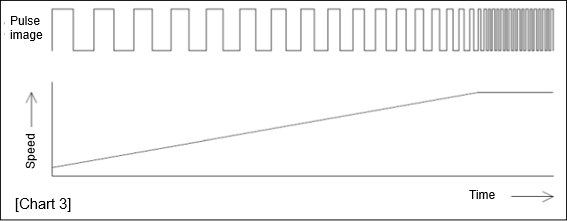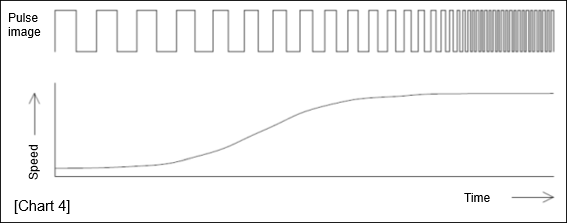# Acceleration and deceleration of stepping motors

This section describes acceleration and deceleration operations when using a stepping motor drive IC. To rotate a stepping motor at high speed, you need to drive it in the pull-out torque range. To drive a stepping motor in the pull-out torque range, it must be accelerated. We will describe acceleration and deceleration operations of a stepping motor from now on.

## 1. Pull-in torque and pull-out torque

“Glossary of terms” in our website says:

Pull-in torque
The maximum torque that a motor can operate at a predetermined pulse frequency and overcome this load to start in the start and stop region.
Also known as starting torque.
Pull-out torque
The maximum torque that a motor can operate synchronously at a specified drive pulse rate in the slewing area. Under load conditions exceeding this pull-out torque, synchronous operation is not available and an out-of-step phenomenon occurs.

The images of pull-in torque and pull-out torque can be explained by driving a car in the more easy-to-understand manner. Please imagine a stick-shift car with a clutch pedal although automatic cars are more popular in recent years.

[Pull-in torque]
To start a car, press the accelerator to increase the velocity of an engine, and connect the clutch. At this time, if the load is too large or you try to start immediately, the car will stall out.
The same can apply to starting a stepping motor. If the load on the shaft is too large or if you try to start suddenly at high speed, the motor cannot start and can go out-of-step.
The pull-in torque characteristic plots the values of revolution and torque that a motor can start without going out-of-step.

[Pull-out torque]
Next, since a car has inertia, once it starts moving, you can use the power required at the start for speed-increase.
However, appropriate engine velocity and shift-ups to match the speed are required for acceleration.
Since a stepping motor also has a moment of inertia, you can use the power required at the start for the rotation speed-increase once the motor starts rotating.
It also requires proper acceleration. The pull-out torque characteristic plots the values of the velocity and torque that a motor can operate without going out-of-step when accelerating.

To rotate a stepping motor at high speed, it should start in the pull-in torque region and accelerate to the pull-out torque region.## 2. Types of acceleration/deceleration

Acceleration of a stepping motor includes “Stepped acceleration/deceleration”, “Linear acceleration/deceleration”, and “S-curve acceleration/deceleration.”

### Stepped acceleration/deceleration

Stepped acceleration/deceleration is to change the speed in step-by-step manner. When operating a drive IC by generating pulses from a microcomputer, etc., the reference pulses may be divided to save the resources of the microcomputer.

For example, if 1000 pps pulse is used as the reference, 100 pps (1/10 of 1000 pps), 200 pps (1/5 of 1000 pps), 333pps (1/3 of 1000 pps), 500 pps (1/2 of 10000 pps), 1000 pps (1/1 of 1000 pps), etc. are created, and these are combined in step-by-step manner to accelerate and decelerate.
However, if the division ratio (denominator) becomes smaller, the speed difference becomes large, therefore, a motor cannot follow the speed changes depending on the load (e.g., increase from 500 pps to 1000 pps).### Linear acceleration/deceleration

Linear acceleration/deceleration is to change the speed linearly, and it can be created by using pulse-generating LSIs (e.g., NPM’s PCL and PCD series.) or microcomputers with sufficient resources.
Linear acceleration/deceleration can perform smoother acceleration/deceleration comparing to stepped acceleration/deceleration, which may be resulting in better motor following.
In addition, when using a pulse generation LSI, the width of the first pulse becomes narrower than the pulse width calculated by the starting speed since the acceleration starts internally at the same time as the operation start.
If you want to start at the starting speed without fail, there is a function called “Idling pulse”, which starts accelerating after operating for several pulses at the starting speed.### S-curve acceleration/deceleration

S-curve acceleration/deceleration is to generate pulses so as to reduce the acceleration rate change at the start of acceleration and at the end of acceleration. It is used to suppress the vibration of the machine and make the movement smoother. You can create S-curve acceleration/deceleration easily by using pulse generate LSIs of NPM or microcomputers with sufficient resources.
The speed curves of S-curve acceleration/deceleration include quadratic functions, sine waves, and cycloid curves (NPM adapts quadratic functions).
However, the characteristics of S-curve acceleration/deceleration may not appear in low speed operations when the difference between starting speed and operating speed is small and the pulse speed changes are small, or when the number of pulses used for acceleration/deceleration is small.## 3. Disturbance Frequency Considerations

Stepping motors have a disturbance frequency which is prone to motor-specific resonance phenomena.
Since the behavior of a motor becomes unstable in this disturbance frequency band, it is necessary to consider the disturbance frequency in acceleration/deceleration as well.

・Avoid disturbance frequencies
For example, if the disturbance frequency band is around 200 pps and there is margin in pull-in torque and a motor can start at higher speed, start at the frequency that avoids the disturbance frequency band (e.g., 300 pps) .

・Accelerate to pass the disturbance frequency in a short time
For example, if the disturbance frequency band is around 200 pps and you want to accelerate from 50 pps to 1000 pps, you should accelerate so as to pass the disturbance frequency band of 200 pps in a short time. Depending on the load and the operating speed to be used, a motor often passes the band within an acceleration/deceleration time of several 10 to 100 ms.

## 4. Move the actual object

We have mainly described accelerations so far. In decelerations, the friction becomes a brake and it may stop a motor in a shorter time than acceleration. In addition, even if pull-in torque and pull-out torque are satisfied in the theoretical calculation, your machine may not move well due to machining errors, assembly errors of the mechanism. Or it may be difficult for you to choose which type of acceleration/deceleration should be performed. In such cases, it is often quicker to actually run the experimental machine or prototype.

We also provide the loan-out service of drivers and controllers, so please feel free to contact us.